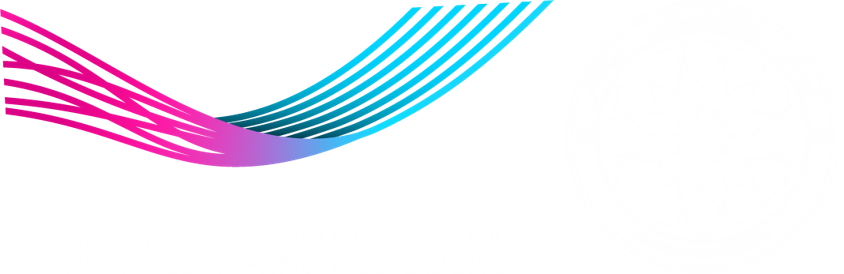# Applied Econometrics

## Applied Econometrics

The goal of this course is to prepare students for using micro-data both for micro and macro applications. I start with estimation methods such as OLS, MLE and GMM then I will cover a number of topics that are important in applied research: Logit, Probit, Hazard models, Cox model, RCTs, IV, DD, RDD, Quintile regression, Propensity Score Matching, linear panel modes and time permitting I will introduce bootstrap methods. Core methods of the course are divided into two categories: structural modeling and reduced form identification methods.

## Lectures

LECTURE 1: INTRODUCTION
LECTURE 2: MICROECONOMICS DATA STRUCTURE
LECTURE 3: ESTIMATION OF LINEAR MODELS
LECTURE 4: MAXIMUM LIKELIHOOD ESTIMATION
LECTURE 5: GMM
LECTURE 6: LOGIT
LECTURE 7: NESTED LOGIT AND GENERAL EXTREME VALUE
LECTURE 8: PROBIT
LECTURE 9: RANDOM UTILITY MODELS WITH THE MIXED LOGIT MODEL
LECTURE 10: TOBIT, SELECTION MODELS AND THE ROY MODEL
LECTURE 11: ECONOMETRICS OF FIELD EXPERIMENTS
LECTURE 12: MACHINE LEARNING AND ECONOMETRICS
LECTURE 13: DURATION ANALYSIS
LECTURE 14: MODELS FOR PANEL DATA
LECTURE 15: CLUSTER ROBUST STANDARD ERRORS
LECTURE 16: ‌BOOTSTRAP METHODS